# 两种修正风力发电机功率曲线的方法研究Two Methods for Correcting the Power Curve of Wind Turbines

DOI: 10.12677/TDET.2018.74009, PDF, HTML, XML, 下载: 717  浏览: 1,890

Abstract: An important indicator for evaluating the performance of wind turbines is the power curve of the wind turbine. In the actual process of fan operation, in order to accurately measure the power curve of the fan, it is necessary to consider the uncertain factors on the site, such as wind direction change, natural wind speed, turbulence intensity, etc., and these uncertain factors bring great difficulties to the measurement. Therefore, in order to obtain the wind turbine power curve simply and accurately, the wind speed correction and air density correction can be performed according to the aerodynamic theory . The wind measured by the corrected wind speed is closer to the wind obtained by the actual unit; by correcting the air density, the density measurement of the on-site air density into standard air is closer to reality. Two corrections of the two methods are used to obtain a more accurate power curve.

1. 引言

2. 本身绘制风力发电机功率曲线的偏差

3. 功率曲线的修正方法

3.1. 修正风速

$W=\frac{1}{2}m{v}^{2}=\frac{1}{2}\left(\rho Avt\right){v}^{2}=\frac{1}{2}\rho A{v}^{3}t$ 

W——风能，单位J；

ρ——空气密度，单位为kg/m3

v——来流速度，单位为m/s；

A——面积，单位为m2

${p}_{w}=W/t=\frac{1}{2}\rho A{v}^{3}$ 

${C}_{p}=\frac{P}{{P}_{0}}=\frac{1}{2}\left(1-{\epsilon }^{2}\right)\left(1+\epsilon \right)$  (1)

${v}^{\prime }=\frac{1}{2}\left({v}_{1}+{v}_{2}\right)$  (2)

P———实测功率；

P0———换算到标准条件下的功率。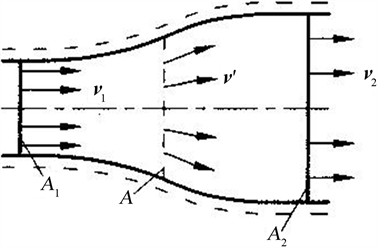v1：风轮前远方风速；v2：风轮后远方风速；v'：气流通过风轮时的风速；A：风轮扫风面积；A1：风轮前扫风面积；A2：风轮后扫风面积

Figure 1. Schematic diagram of airflow in a wind turbine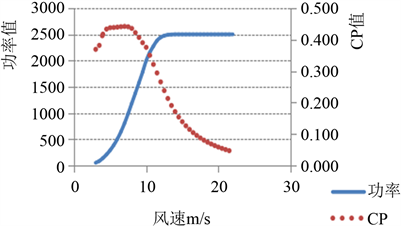Figure 2. Relationship between wind speed and power factor CP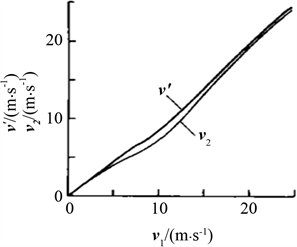Figure 3. Relationship between front and rear wind turbine speed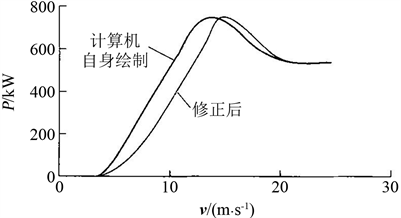Figure 4. Relationship between 1.5 KW horizontal axis wind turbine parameters 

3.2. 修正空气密度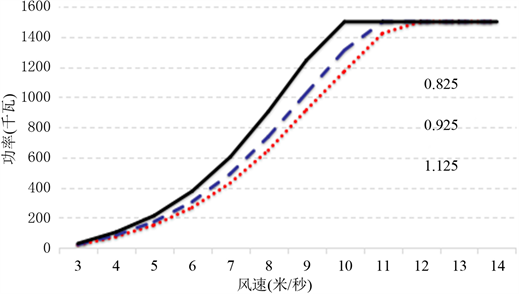Figure 5. Comparison of wind turbine power curves at different air densities

$\rho ={\rho }_{0}\frac{{T}_{0}}{T}×\frac{P}{{P}_{0}}$ 

${P}_{0}=\frac{{\rho }_{0}}{\rho }P$ 

P——在现场轮毂高度处测量的大气压力功率曲线经过第二次修正，其精确度和可比性大大提高。

4. 对比验证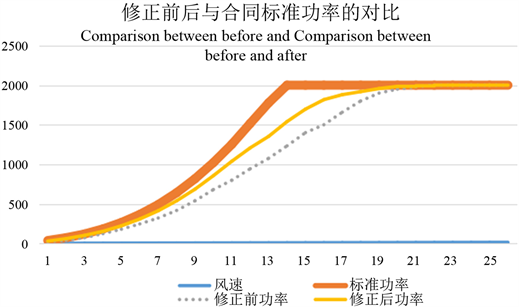Figure 6. Comparison of standard power with correction by two methods

5. 结束语

   王承煦, 张源. 风力发电[M]. 北京: 中国电力出版社, 2003.  谢建民, 邱毓昌, 张治源. 风力发电机优化选型与云南省风力发电厂规划研究[J]. 电力建设, 2001, 22(5): 27-31.  王珊珊. 风力发电技术现状及发展趋势[J]. 电子技术与软件工程, 2017(4): 238.  Jangamshetti, S.H. and Rau, V.G. (1999) Site Matching of Wind Tur-bine Generators: A Case Study. IEEE Transactions on Energy Conversion, 14, 1537-1543. https://doi.org/10.1109/60.815102